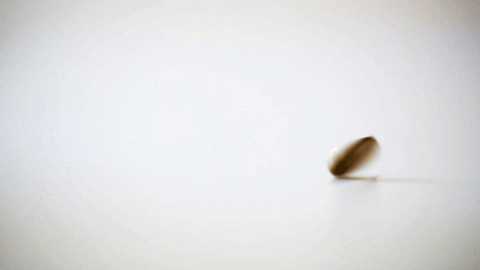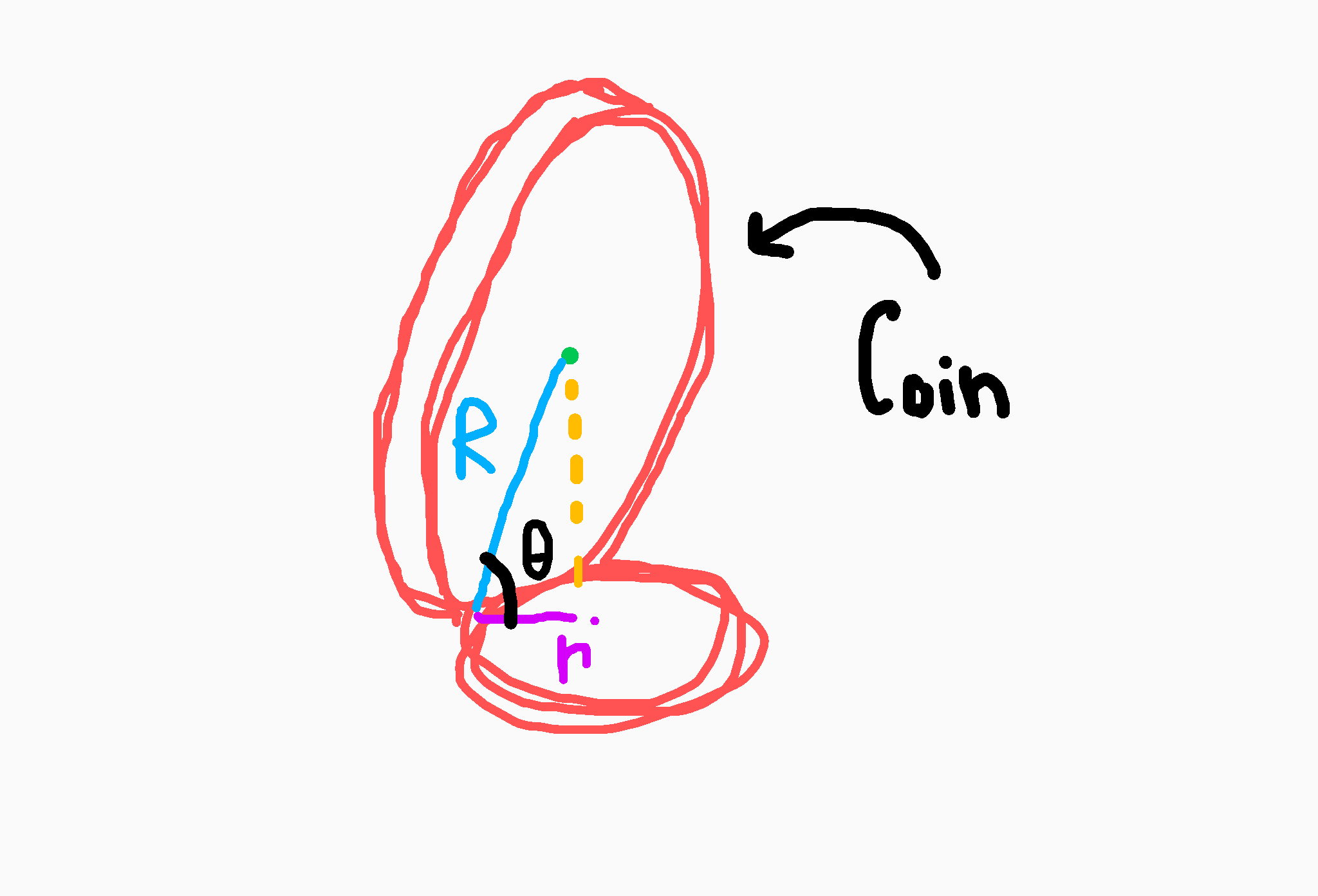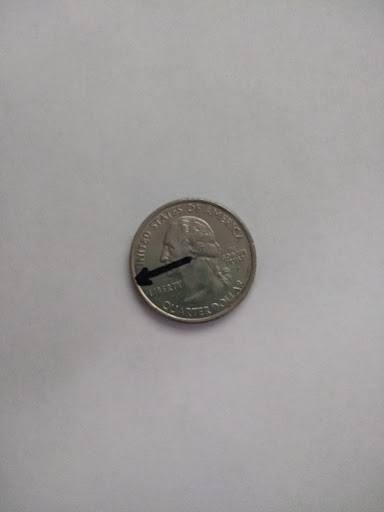Coins! As some of you may relate to this, I love to take a coin, and just spin it on a flat surface or table. It's just satisfying, but being put to shame by the so called "Fidget Spinner". I was spinning a coin a few days ago, and was ultimately bored at the time, so I decided to ask myself a simple question: What information from the coin can be taken away from it spinning? This then was taken into is there a correlation, or ratio, between the rate at which the coin rotates, and the rate at which it "wobbles". With a goal in mind, I picked up my pencil and started to work things out.

First things first, defining and finding all of our givens:This diagram represents what the coin might look like at a given instance.

What we know about the coin:

The radius of our coin $= R$
The circumference of our coin $C = 2 \pi R$

Now for the circle our coin rotates and wobbles upon:

The radius of this circle is entirely dependant on the angle of the coin to the horizontal (table/flat surface. We will define that as $\theta$). Using the diagram, we can find that $r = R \cos (\theta)$
The circumference is $c = 2 \pi R \cos (\theta)$

With all the givens that we need out of the way, on to the application.

A thing to note is that as the coin completes a full rotation around the smaller circle, the original placement of the coin moves by a certain amount. You can easily demonstrate this by drawing an arrow on a quarter, and guiding it through a rotation on a circle smaller than the quarter.This was the quarter I used when getting ideas on how the coin behaved.

This extra distance it covers can easily be thought out to be as $C - c$.

$C - c = 2 \pi R - 2 \pi R \cos (\theta) = 2 \pi R (1 - \cos(\theta))$

$2 \pi R (1 - \cos(\theta)) =$ the rate at which the distance our coin completes per revolution.

The rate for the distance per revolution our coin completes while "wobbling" is the same as the circumference our coin moves around upon, which we know is $2 \pi R \cos(\theta)$

Putting these two together as ratio of rate of rotation, to rate of "wobbling", we get:

$\frac{\textrm{Rate of Spinning}}{\textrm{Rate of Wobbling}} = \frac{2 \pi R \cos (1 - \theta)}{2 \pi R cos (\theta)}$

$\large= \frac{1}{\cos (\theta)} - 1$

This expression represents that at any given moment, the ratio between how fast the coin is spinning, and the how fast the coin is "wobbling" (which can be seen as the amount of hertz produced by the coin), will be $\frac{1}{\cos (\theta)} - 1$. This also means that if you multiply the frequency of "wobbling" by this expression, it will output how fast the coin should be spinning at the given value of theta. For example, let's say the coin is wobbling at a frequency of $5\,hertz$ at an angle of $\frac{\pi}{4}\,radians$ (because radians are cool), the coin would have to be rotating about $4.52\,revolutions\,a\,second$ to maintain that angle to the horizontal at that "wobbling" frequency (because of how hertz measure $cycles\,per\,second$, the cycles translate into revolutions for the output).

Of course, this is all theoretical. In practice, the coin may slip. Wind may change the local air pressure, thus changing the air resistance. Everything needs to stay constant, with no disturbances or changes occuring during the coin's movement. But this is still a neat thing if you were to ask me!

Just to recap, we took the basics and givens of our coin and it's enviornment. Used those to get the generalized rates of the coin spinning and wobbling. We then used those calculate our ratio between the two at a given instant. Not bad!